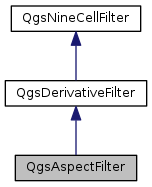QgsAspectFilter Class Reference

Calculates aspect values in a window of 3x3 cells based on first order derivatives in x- and y- directions. More...

`#include <qgsaspectfilter.h>`

Inheritance diagram for QgsAspectFilter:[legend]

## Public Member Functions

QgsAspectFilter (const QString &inputFile, const QString &outputFile, const QString &outputFormat)

float processNineCellWindow (float *x11, float *x21, float *x31, float *x12, float *x22, float *x32, float *x13, float *x23, float *x33) override
Calculates output value from nine input values. More...Public Member Functions inherited from QgsDerivativeFilter
QgsDerivativeFilter (const QString &inputFile, const QString &outputFile, const QString &outputFormat)Public Member Functions inherited from QgsNineCellFilter
QgsNineCellFilter (const QString &inputFile, const QString &outputFile, const QString &outputFormat)
Constructor that takes input file, output file and output format (GDAL string) More...

virtual ~QgsNineCellFilter ()=default

double cellSizeX () const

double cellSizeY () const

double inputNodataValue () const

double outputNodataValue () const

int processRaster (QgsFeedback *feedback=nullptr)
Starts the calculation, reads from mInputFile and stores the result in mOutputFile. More...

void setCellSizeX (double size)

void setCellSizeY (double size)

void setInputNodataValue (double value)

void setOutputNodataValue (double value)

void setZFactor (double factor)

double zFactor () constProtected Member Functions inherited from QgsDerivativeFilter
float calcFirstDerX (float *x11, float *x21, float *x31, float *x12, float *x22, float *x32, float *x13, float *x23, float *x33)
Calculates the first order derivative in x-direction according to Horn (1981) More...

float calcFirstDerY (float *x11, float *x21, float *x31, float *x12, float *x22, float *x32, float *x13, float *x23, float *x33)
Calculates the first order derivative in y-direction according to Horn (1981) More...Protected Attributes inherited from QgsNineCellFilter
double mCellSizeX = -1.0

double mCellSizeY = -1.0

QString mInputFile

float mInputNodataValue = -1.0
The nodata value of the input layer. More...

QString mOutputFile

QString mOutputFormat

float mOutputNodataValue = -1.0
The nodata value of the output layer. More...

double mZFactor = 1.0
Scale factor for z-value if x-/y- units are different to z-units (111120 for degree->meters and 370400 for degree->feet) More...

## Detailed Description

Calculates aspect values in a window of 3x3 cells based on first order derivatives in x- and y- directions.

Direction is clockwise starting from north

Definition at line 27 of file qgsaspectfilter.h.

## Constructor & Destructor Documentation

 QgsAspectFilter::QgsAspectFilter ( const QString & inputFile, const QString & outputFile, const QString & outputFormat )

Definition at line 21 of file qgsaspectfilter.cpp.

## Member Function Documentation

 float QgsAspectFilter::processNineCellWindow ( float * x11, float * x21, float * x31, float * x12, float * x22, float * x32, float * x13, float * x23, float * x33 )
overridevirtual

Calculates output value from nine input values.

The input values and the output value can be equal to the nodata value if not present or outside of the border. Must be implemented by subclasses

Implements QgsDerivativeFilter.

Definition at line 27 of file qgsaspectfilter.cpp.

The documentation for this class was generated from the following files: